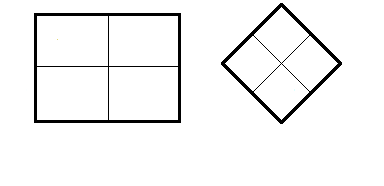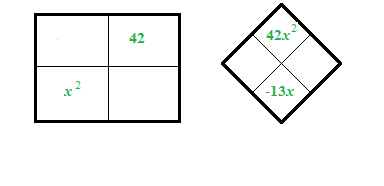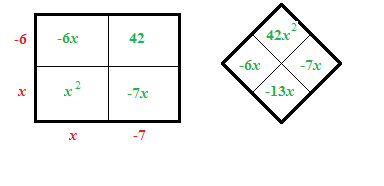### Home > CCA > Chapter 9 > Lesson 9.1.2 > Problem9-17

9-17.

Solve the following quadratic equations by factoring and using the Zero Product Property. Be sure to check your solutions.

1. $x^2-13x+42=0$

Draw a generic rectangle and diamond. Draw a rectangle and divide it into four equal parts. Blank Diamond Problem.

Factor. Added to the rectangle: Interior upper right box is negative 42. Interior lower left box is X squared.  Added to the diamond problem: Left blank, Right blank, Top 42, x squared, Bottom negative 13, x.

$(x−6)(x−7)=0$  Added to the rectangle: Interior upper left box is negative 6, x. Interior lower right box is negative 7, X. Exterior left edge top is negative 6. Exterior left edge bottom is X. Exterior bottom edge left is X. Exterior bottom edge right is negative 7.  Added to the diamond problem: Left negative 6, x, Right negative 7, x.

One solution is $x=6$.
Make sure you find the other solution. Added to the diamond problem: Left negative 6, x, Right negative 7, x.1. $0=3x^2+10x-8$

$0=\left(3x-2\right)\left(?\ +\ ?\right)$

1. $2x^2-10x=0$

There are only $2$ terms, so factor using the GCF.

1. $4x^2+8x-60=0$

Notice that each term is a multiple of $4$.

$x=3$ or $x=-5$